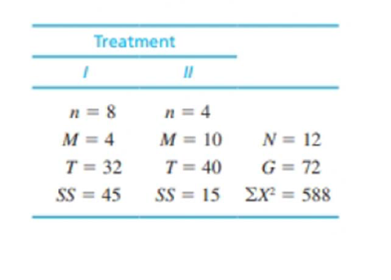Chapter 12, Problem 20PEssentials of Statistics for the B...

8th Edition
Frederick J Gravetter + 1 other
ISBN: 9781133956570

Solutions

Chapter
SectionEssentials of Statistics for the B...

8th Edition
Frederick J Gravetter + 1 other
ISBN: 9781133956570
Textbook Problem

The following values summarize the results from an independent-measures study comparing two treatment conditions.a. Use an independent-measures t test with α = .05 to determine whether there is a significant mean difference between the two treatments. You should find that F = t2.b. Use an ANOVA with α = .05 to determine whether there is a significant mean difference between the two treatments.a.

To determine
Whether there in a significant mean difference between the two treatments using t test.

Explanation

Given info:

From a study of independent measures for comparing two treatments,

 Treatment 1 Treatment 2 N=12G=72∑X2=588 n=8 n=4 M=4 M=10 T=32 T=40 SS=45 SS=15

Calculation:

The null and alternative hypotheses:

Null hypothesis:

H0:There is no significant difference between the two treatments

Alternate hypothesis:

Ha:There is significant different between the two treatments

Degrees of freedom:

It is known that for k number of treatments the degrees of freedom is,

df=n1+n22.

Thus,

df=n1+n22=8+42=10

Critical value:

From t-distribution table, with α=0.05 and df=10, the critical value is ±2.228

Pooled variance:

The pooled variance is sp2=SS1+SS2df1+df2.

Thus,

sp2=SS1+SS2df1+df2=45+157+3=6

Estimated standard error:

The estimated standard error is sM1-M2=sp2n1+sp2n2

b.

To determine
Whether there is a significant mean difference between the two treatments using ANOVA at α=0.05.

To check: Fratio=t2

Still sussing out bartleby?

Check out a sample textbook solution.

See a sample solution

The Solution to Your Study Problems

Bartleby provides explanations to thousands of textbook problems written by our experts, many with advanced degrees!

Get Started

Find more solutions based on key concepts